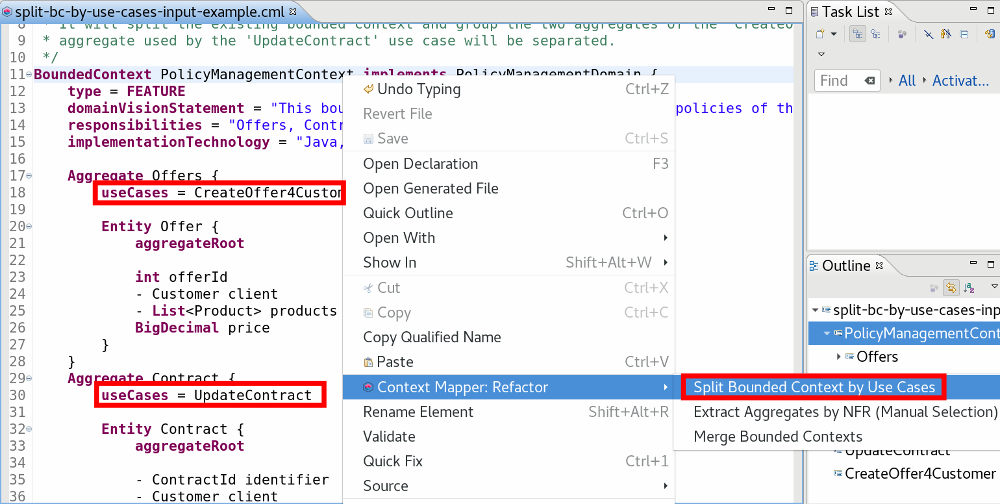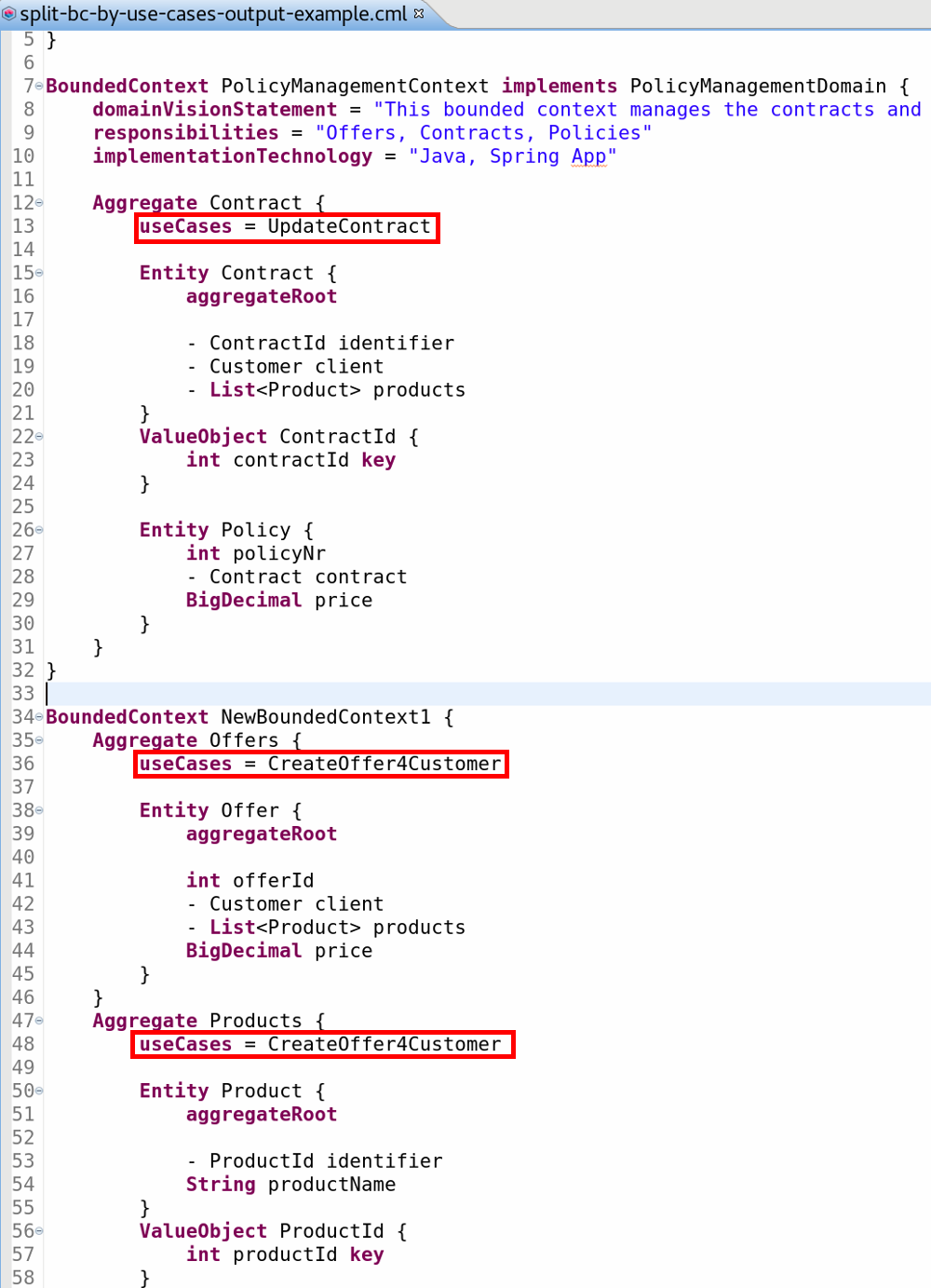# AR-2: Split Bounded Context by Use Cases

Splits a bounded context by grouping those aggregates together into one bounded context which are used by the same use case(s).

Hint: An aggregate in CML can belong to multiple use cases (therefore the plural use cases in the AR name).

## Context & Rationales

By decomposing a system into multiple bounded contexts we aim for loose coupling between the bounded context and a high cohesion within them. One approach to achieve this and to decompose a system into components or (micro-) services is to split by use cases.

In Context Mapper you can assign multiple use cases to an aggregate, which allows you to model by which use cases an aggregate is used. Consult our aggregate documentation page to see how this can be modeled in CML.

## Goal

This Architectural Refactoring (AR) splits a bounded context by use cases. This means, it creates bounded contexts containing aggregates which are used by the same use cases. It can be applied if your model exhibits a bounded contexts with aggregates which are used by multiple different use cases.

Inverse AR’s:

## Preconditions

• The bounded context must contain at least two aggregates.
• The aggregates must be assigned to different use cases.

## Input

• One bounded context.

## Output

• The AR creates multiple bounded contexts. Each bounded context contains one or more aggregates which are used by the same use cases.

## Example

The following example illustrates how this AR can be applied. The corresponding sources can be found in our examples repository.

### Input

The example bounded context contains two aggregates which are used by different use cases. The AR is available on the bounded context:### Result

The resulting model contains two bounded contexts, one for each use case:## Example Sources

• You can find the CML sources for this AR example here.